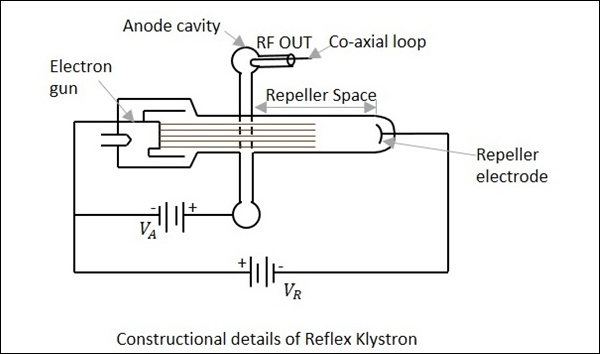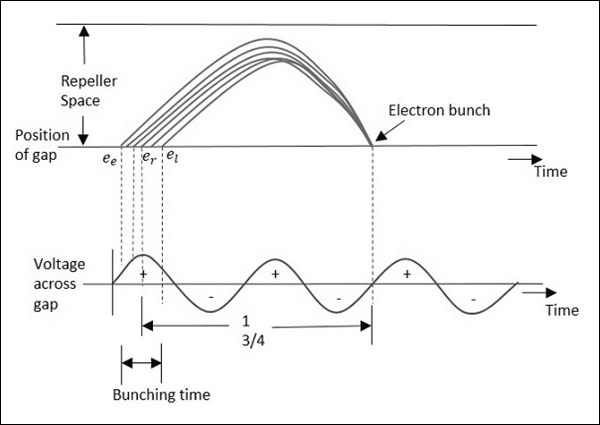# Microwave Engineering - Reflex Klystron

This microwave generator, is a Klystron that works on reflections and oscillations in a single cavity, which has a variable frequency.

Reflex Klystron consists of an electron gun, a cathode filament, an anode cavity, and an electrode at the cathode potential. It provides low power and has low efficiency.

## Construction of Reflex Klystron

The electron gun emits the electron beam, which passes through the gap in the anode cavity. These electrons travel towards the Repeller electrode, which is at high negative potential. Due to the high negative field, the electrons repel back to the anode cavity. In their return journey, the electrons give more energy to the gap and these oscillations are sustained. The constructional details of this reflex klystron is as shown in the following figure.It is assumed that oscillations already exist in the tube and they are sustained by its operation. The electrons while passing through the anode cavity, gain some velocity.

## Operation of Reflex Klystron

The operation of Reflex Klystron is understood by some assumptions. The electron beam is accelerated towards the anode cavity.

Let us assume that a reference electron er crosses the anode cavity but has no extra velocity and it repels back after reaching the Repeller electrode, with the same velocity. Another electron, let's say ee which has started earlier than this reference electron, reaches the Repeller first, but returns slowly, reaching at the same time as the reference electron.

We have another electron, the late electron el, which starts later than both er and ee, however, it moves with greater velocity while returning back, reaching at the same time as er and ee.

Now, these three electrons, namely er, ee and el reach the gap at the same time, forming an electron bunch. This travel time is called as transit time, which should have an optimum value. The following figure illustrates this.The anode cavity accelerates the electrons while going and gains their energy by retarding them during the return journey. When the gap voltage is at maximum positive, this lets the maximum negative electrons to retard.

The optimum transit time is represented as

$$T = n + \frac{3}{4} \quad where \: n \: is \:an \:integer$$

This transit time depends upon the Repeller and anode voltages.

## Applications of Reflex Klystron

Reflex Klystron is used in applications where variable frequency is desirable, such as −